# Vibrating Sample Magnetometer (VSM)

1488 words (6 pages) Essay in Physics

12/03/18 Physics Reference this

Disclaimer: This work has been submitted by a student. This is not an example of the work produced by our Essay Writing Service. You can view samples of our professional work here.

Any opinions, findings, conclusions or recommendations expressed in this material are those of the authors and do not necessarily reflect the views of UK Essays.

• Robert Konstandelos

Operation

A sample is made to oscillate using a vibrational unit extended on a rod. The sample is placed between two electromagnetic pieces which are used as the applied field for this this experiment. With the sample oscillating induces a voltage between the search coils which creates a signal to determine the magnetic properties of the sample. Reference coils are used to create a reference signal such that noise generated from the signal can be filtered using a lock-in amplifier . Because the signal and the reference signal are directly related through its voltage and amplitude means that precise measurements can be recorded using a voltmeter. Calibration methods are important to determine the relation between the voltages induced by the magnetic field and the sample and their magnetic properties. Calibrating the applied field is done by increasing the voltage in steps measuring the field until reaching a maximum. The system is calibrated using a nickel standard normally as a number of volts per unit of magnetic moment. Many materials such as types of barium ferrite or alnico materials can be placed inside to determine properties. These properties include remanence, coercivity, intrinsic coercivity and operating points once the system has been calibrated.

Advantages and Disadvantages in terms of experimental facets

The key advantage is the precision and accuracy of VSMs. Taking measurements at a range of angles once detection arrangements for the coils have been devised can be done. The advantage of sample vibration perpendicularly to the applied field can be found once the detection coils have been arranged appropriately. This means that there is the ability to test the sample at different angles. The positioning of the coils are done in a way to reduce the effects of sample position variation and external field variation- essentially deep into the applied field shown in figure 1. Disadvantages are that they are not well suited for determining the magnetisation loop or the hysteresis curve due to the demagnetising effects of the sample. Another problem is that, particularly for the VSM used in the third year laboratory is that temperature dependence cannot be controlled.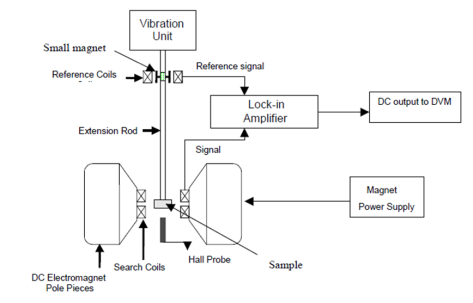Figure 1. A schematic layout of the VSM

2. B-H Hysteresis Loop Tracer

Operation

The B-H hysteresis loop tracer is essentially two coils, one with a sample and the other which is empty for comparison. The insertion of a sample into the pickup coils causes a voltage proportional to the rate of change of the vector field to occur across the difference amplifier. After passing through an integrator, a voltage proportional to the intrinsic induction is passed to the Y-amp of the oscilloscope. This voltage combined with an X-voltage representing the magnetising field generated from the solenoid without the sample results in the generation of a hysteresis loop on the oscilloscope. Calibration is through a balance and phase adjustment to establish a trace on the oscilloscope. They are done to make sure that the magnetising field is linear and that every vector corresponds to the applied field. Measurements for the magnetic properties can then be made.

Advantages and disadvantages in terms of experimental facets

The coils have the ability to heat the sample such that temperature variance can be observed in the way that the material behaves when influenced by a magnetic field. On the other hand, this could cause overheating of the system which could result in a failure. Using a BH-looper can give the user a more improved visualisation compared to a VSM of the way a material behaves. The values plotted on the scope are only proportional to the absolute values, therefore display yields qualitative not quantitative information about a material magnetic properties. The precision is generally low compared to a VSM. Because a hysteresis loop is viewed using an oscilloscope means that observations of whether the material is a soft or hard magnetic material. And this is why it is used in quality control testing industries like the control of ferromagnetic oxides in a magnetic tape factory.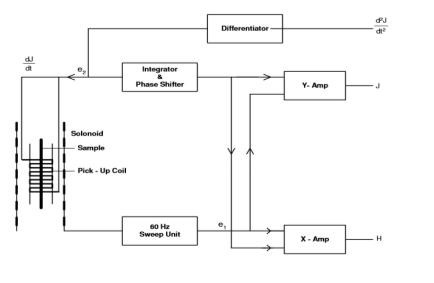Figure 2. A schematic layout of a BH loop tracer .

3(I) Difference between concepts of Vector Field B, Magnetisation M and the magnetising field H

The vector field B represents the magnetic induction. Magnetisation M is the magnetic moment per unit volume of a solid. Magnetising H field is the magnetic field strength. These three quantities are related by the equation.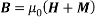With μ0 being the permittivity of free space. To show the difference between these quantities, hysteresis loops for a magnetic material shown in figure 4 are used. One of the key differences shown is that the magnetisation saturates whereas the B field increases at a constant rate for certain values for H. The magnetisation is generated by the spin and the orbital angular momentum of electrons in the solid. H is generated outside the material by electrical currents. Therefore, from the equation above, the B field is the combination of H and M which shows the difference between the quantities with the inclusion of the permittivity of free space.

A way to show the difference between the 3 parameters is through the representation of a bar magnet in a magnetic field shown in figure 3. Unfortunately, due to the age of the diagram, the labels are a bit old. Hence the ‘True’ field denotes the vector field B and the Applied field represents the magnetisation M. However, the arrows represent the direction and strength of each parameter. It is clear from figure 3 that the Magnetisation is much stronger than the demagnetising field.Figure 3 An example of a magnet being demagnetised in an applied field

From figure 4, the two sketches representing of B and M against H can give an understanding of other magnetic properties of the material. The curve on the left can show the saturation of the magnetic material as well as the remanence Mr– the remaining magnetisation after the applied field has been turned off. The right hand diagram can show the remanent induction Br and the saturation point of the applied field. In terms of the difference between the parameters, M, B and H, they yield different properties of the material in question.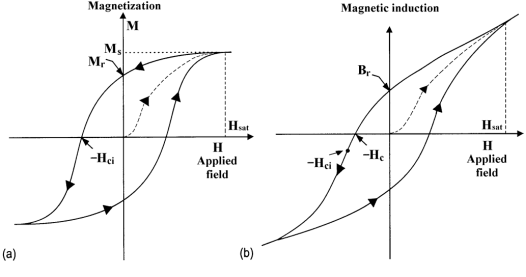Figure 4 Hysteresis loops showing (a) M and (b) B field against H

3(II) The difference between the susceptibility and relative permeability

The relative permeability μr and susceptibility χ are very closely related as shown by the equation below: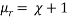The relative permeability represents a characterisation of magnetic materials. Paramagnetic or diamagnetic materials have permeabilities close to the permeability of free space. However for ferromagnetic materials, the permeability is large in comparison. It represents a multiplication factor. For example, the use of an iron core with a relative permeability is 200 times greater than just an air coil used. So this is a measure of the actual magnetic field within a ferromagnetic material. Susceptibility is a measure to an extent to which a material may be magnetised in a magnetic field. It represents a ratio of how much a material is magnetised compared to the applied field on that material . So the susceptibility specifies how much the relative permeability differs from one as shown in the equation above.

References

 Foner S 1959 Versatile and Sensitive Vibrating-Sample Magnetometer* Rev. Sci. Instrum. 30 548–57

 Howling D H 1956 Simple 60-cps Hysteresis Loop Tracer for Magnetic Materials of High or Low Permeability Rev. Sci. Instrum. 27 952

 Jiles D 1990 Introduction to Magnetism and Magnetic Materials (Chapman and Hall)

 Magnetic Susceptibilty http://www.britannica.com/EBchecked/topic/357313/magnetic-susceptibility

#### Cite This Work

To export a reference to this article please select a referencing stye below:

## Related Services

View all

### DMCA / Removal Request

If you are the original writer of this essay and no longer wish to have the essay published on the UK Essays website then please:Prices from
###### £28

Undergraduate 2:2 • 250 words • 7 day delivery

Delivered on-time or your money back

##### Rated 4.6 out of 5 by(139 Reviews)